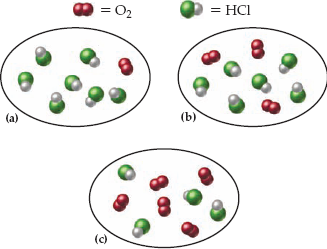×
Get Full Access to Introductory Chemistry - 5 Edition - Chapter 8 - Problem 53p
Get Full Access to Introductory Chemistry - 5 Edition - Chapter 8 - Problem 53p

×

# Consider the reaction:4 HCl(g) + O2(g) ? 2 H2O(g) + 2 Cl2(g)Each molecular diagramISBN: 9780321910295 34

## Solution for problem 53P Chapter 8

Introductory Chemistry | 5th Edition

• Textbook Solutions
• 2901 Step-by-step solutions solved by professors and subject experts
• Get 24/7 help from StudySoup virtual teaching assistantsIntroductory Chemistry | 5th Edition

4 5 1 427 Reviews
19
4
Problem 53P

PROBLEM 53P

Consider the reaction:

4 HCl(g) + O2(g) → 2 H2O(g) + 2 Cl2(g)

Each molecular diagram represents an initial mixture of the reactants. How many molecules of Cl2 are formed by complete reaction in each case? (Assume 100% actual yield.)Step-by-Step Solution:

Problem 53P

Consider the reaction:Each molecular diagram represents an initial mixture of the reactants. How many molecules of Cl2 are formed by complete reaction in each case? (Assume 100% actual yield.)Step by step solution

Step 1 of 3

a)

From the given,

The balanced chemical reaction is as follows.The actual yield is 100%.

From the balanced chemical reaction, four moles of HCl reacts with one mole of oxygen to produce two moles of chlorine.

Let’s consider the Figure -1:

There are seven moles of  HCl and one mole of oxygen .

Let’s calculate the moles ofproduced by using above reactants.If HCl is limiting reactant 3.5 moles of chlorine is produced.

From the balanced chemical reaction, one mole of oxygen gives two moles of chlorine.

In the given picture one mole of oxygen is present. So, it gives two moles of chlorine.

Hence, oxygen produces less amount of product .So, it is a limiting reactant.

Therefore, one mole of oxygen produces 2 moles of chlorine.

__________________________________________________________________________

Step 2 of 3

Step 3 of 3

##### ISBN: 9780321910295

Since the solution to 53P from 8 chapter was answered, more than 721 students have viewed the full step-by-step answer. Introductory Chemistry was written by and is associated to the ISBN: 9780321910295. This full solution covers the following key subjects: reaction, initial, Case, complete, consider. This expansive textbook survival guide covers 19 chapters, and 2046 solutions. The answer to “Consider the reaction:4 HCl(g) + O2(g) ? 2 H2O(g) + 2 Cl2(g)Each molecular diagram represents an initial mixture of the reactants. How many molecules of Cl2 are formed by complete reaction in each case? (Assume 100% actual yield.)” is broken down into a number of easy to follow steps, and 38 words. This textbook survival guide was created for the textbook: Introductory Chemistry, edition: 5. The full step-by-step solution to problem: 53P from chapter: 8 was answered by , our top Chemistry solution expert on 05/06/17, 06:45PM.

Unlock Textbook Solution